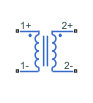# Mutual Inductor

Mutual inductor in electrical systems

## Library

Electrical Elements

•## Description

The Mutual Inductor block models a mutual inductor, described with the following equations:

`$V1=L1\frac{dI1}{dt}+M\frac{dI2}{dt}$`
`$V2=L2\frac{dI2}{dt}+M\frac{dI1}{dt}$`
`$M=k\sqrt{L1·L2}$`

where

 `V1` Voltage across winding 1 `V2` Voltage across winding 2 `I1` Current flowing into the + terminal of winding 1 `I2` Current flowing into the + terminal of winding 2 `L1`, `L2` Winding self-inductances `M` Mutual inductance `k` Coefficient of coupling, 0 < `k` < 1 `t` Time

This block can be used to represent an AC transformer. If inductance and mutual inductance terms are not important in a model, or are unknown, you can use the Ideal Transformer block instead.

The two electrical networks connected to the primary and secondary windings must each have their own Electrical Reference block.

### Variables

To set the priority and initial target values for the block variables prior to simulation, use the Initial Targets section in the block dialog box or Property Inspector. For more information, see Set Priority and Initial Target for Block Variables.

Nominal values provide a way to specify the expected magnitude of a variable in a model. Using system scaling based on nominal values increases the simulation robustness. Nominal values can come from different sources, one of which is the Nominal Values section in the block dialog box or Property Inspector. For more information, see Modify Nominal Values for a Block Variable.

## Parameters

Inductance L1

Self-inductance of the first winding. The default value is `10` H.

Inductance L2

Self-inductance of the second winding. The default value is `0.1` H.

Coefficient of coupling

Coefficient of coupling, which defines the mutual inductance. The parameter value should be greater than zero and less than 1. The default value is `0.9`.

## Ports

The block has four electrical conserving ports. Polarity is indicated by the + and – signs. Ports labeled 1+ and 1– are connected to the primary winding. Ports labeled 2+ and 2– are connected to the secondary winding.

## Version History

Introduced in R2007a